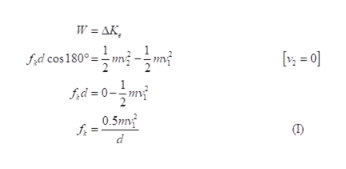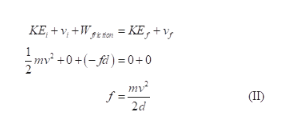# A 7.80-g bullet moving at 520 m/s penetrates a tree trunk to a depth of 5.30 cm.(a) Use work and energy considerations to find the average frictional force that stops the bullet. (b) Assuming the frictional force is constant, determine how much time elapses between the moment the bullet enters the tree and the moment it stops moving.

Question
4 views

A 7.80-g bullet moving at 520 m/s penetrates a tree trunk to a depth of 5.30 cm.

(a) Use work and energy considerations to find the average frictional force that stops the bullet.

(b) Assuming the frictional force is constant, determine how much time elapses between the moment the bullet enters the tree and the moment it stops moving.

check_circle

Step 1

a)

Write the mathematical expression for work energy theorem.help_outlineImage TranscriptioncloseW = AK, [: = 0] fd cos 180° = 0.5mv f =- (1) fullscreen
Step 2

Substitute the values in equation (I).

Step 3

According to the conserv...help_outlineImage TranscriptioncloseKE, + v, +W geton = KE,+ v; mv +0+(-fi ) =0+0 mv? f = 2d (II) fullscreen

### Want to see the full answer?

See Solution

#### Want to see this answer and more?

Solutions are written by subject experts who are available 24/7. Questions are typically answered within 1 hour.*

See Solution
*Response times may vary by subject and question.
Tagged in

### Science